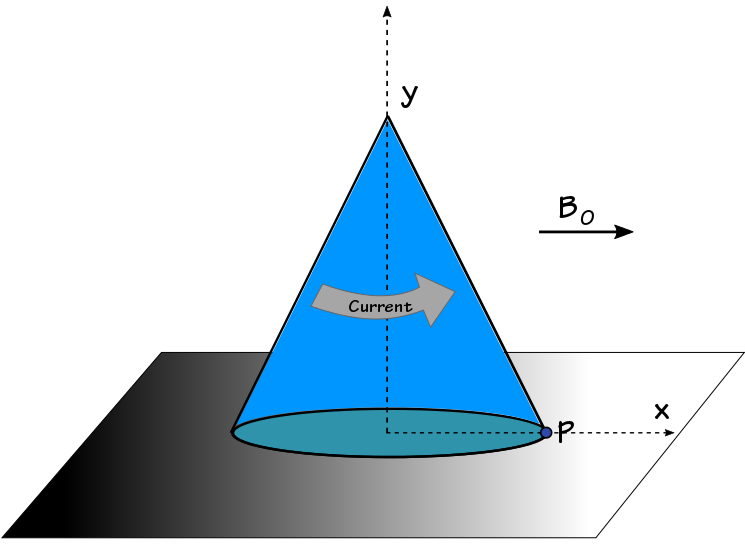# Current round Cone!A cone $($mass $m$, radius $R$, and height $h)$ has a current $I$ flowing uniformly along its curved surface area. This cone is kept on a rough surface in a magnetic field $\vec{B} = B_{0} \hat{i}$.

If the maximum value of current such that the cone doesn't topple about point $P$ is $\dfrac{nmg}{\pi B_{0} R},$ determine the value of $n$.

×